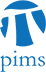## DG-MP-PDE Seminar: Branched transport problems and elliptic approximation

• Date: 06/30/2010
Lecturer(s):
Filippo Santambrogio (Université Paris-Dauphine)
Location:

University of British Columbia

Topic:

Branched transport problems and elliptic approximation

Description:

The branched transport problem is the minimization of a concave functional on vecror measures with prescribed divergence. The only admissible measures are those concentrated on 1-rectifiable sets and the energy is the integral of a power $\theta^\alpha$ of their multiplicity $\theta$. I'll present an approximation by Gamma-convergence, through elliptic functionals defined on more regular functions : the idea is minimizing fucntionals such as $\frac 1 \varepsilon \int |v|^\alpha + \varepsilon |Dv|^2$ under constraints on the divergence of the $H^1$ function $v$. Obviously the exponents on the $\varepsilon$ and on the power of $|v|$ are to be changed if the result wants to be true. This approximation result recalls those of Modica-Mortola for the perimeter functional, where a double-well potential $W$, minimal on $0$ and $1$, is considered, and the energies $\frac 1 \varepsilon \int W(v)+ \varepsilon |Dv|^2$ converge to the perimeter of the interface between $\{v=0\}$ and $\{v=0\}$. Here the double-well is replaced with a concave power, so that there is a sort of double-well at $0$ and $\infty$. In ths case as well, the energy at the limit concentrates on a lower dimensional structure. Besides the link with the theory of elliptic approximations, the interest of this convergence lies in its applications for numerics. Actually, we built (in collaboration with E. Oudet, Chambéry) a quite efficient method, which allows to find reasonable local minima of the limit problem, avoiding the NP complications of the usual combinatorial approaches. The Steiner problem of minimal connection may be approached in this way as well.

Schedule:

3:00 - 4:00pm, WMAX 110.